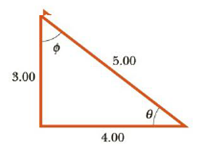Chapter 1, Problem 49P

Chapter
Section
Textbook Problem

In Figure P1.49, find (a) the side opposite θ, (b) the side adjacent to Φ. (c) cos θ, (d) sin Φ, and (c) tan Φ.Figure P1.49

(a)

To determine
The side opposite length of the angle θ .

Explanation

The diagram for the triangle is

From figure, the opposite side of the angle θ is 3unit .

Thus, the opposite side (c)

(b)

To determine
The side adjacent length of the angle ϕ .

(c)

To determine
The cosine of the angle θ .

(d)

To determine
The sine of the angle ϕ .

(e)

To determine
The tan of the angle ϕ .

Still sussing out bartleby?

Check out a sample textbook solution.

See a sample solution

The Solution to Your Study Problems

Bartleby provides explanations to thousands of textbook problems written by our experts, many with advanced degrees!

Get Started

To overload a muscle is never productive. T F

Nutrition: Concepts and Controversies - Standalone book (MindTap Course List)

What is population genetics?

Human Heredity: Principles and Issues (MindTap Course List)

Find the equivalent resistance between points a and b in Figure P27.33. Figure P27.33

Physics for Scientists and Engineers, Technology Update (No access codes included)